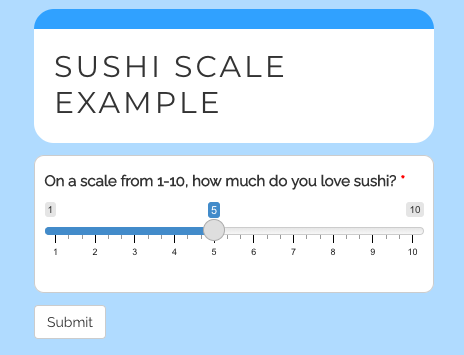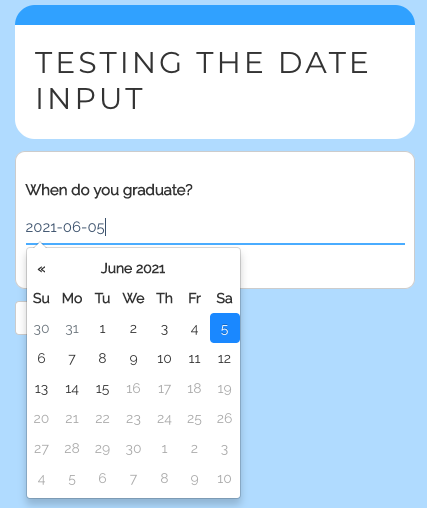Note: This is a pared down version of an article originally posted on my personal blog. I plan to update documentation there more regularly than in the vignettes.

Motivation

The original idea for {shinysurveys} was to provide a select set of well-supported input types that are commonly used with surveys. However, shortly after the package was published on CRAN, additional input types were requested (see GitHub issue #6 or #18).

In order to make the package as light-weight as possible (minimize dependence on external code), I did not wish to implement any input types not native to {shiny}. I also did not want to rewrite the internals of {shinysurveys} whenever a new input-type was requested. As a solution, I developed a framework for custom inputs to allow users to include different input types that meet their use case. In the next section, I outline two examples of how to add custom input types.

Examples

Consider the question “On a scale from 1-10, how much do you love sushi?”. An ideal input type would be {shiny}’s sliderInput. However, this is not natively supported by {shinysurveys} as the slider input requires multiple arguments, including a minimum, maximum, and starting value. To get around this, we can define a new input type using a new function extendInputType(). As in a typical shiny survey, we can define our question as follows:

# Define a question as normal with the input_type set to "slider", which is not natively supported by {shinysurveys}.

slider_question <- data.frame(
question = "On a scale from 1-10, how much do you love sushi?",
option = NA,
input_type = "slider",
input_id = "sushi_scale",
dependence = NA,
dependence_value = NA,
required = TRUE
)

This looks like:

#>                                            question option input_type
#> 1 On a scale from 1-10, how much do you love sushi?     NA     slider
#>      input_id dependence dependence_value required
#> 1 sushi_scale         NA               NA     TRUE

If we try to define the user-interface component of the shiny application, we will get the following error which most commonly occurs when {shinysurveys} doesn’t recognize an input type.

library(shiny)
library(shinysurveys)

ui <- fluidPage(
surveyOutput(df = slider_question,
survey_title = "Testing the Slider Input")
)
#> Error in FUN(X[[i]], ...): Input type 'slider' from the supplied data frame of questions is not recognized by {shinysurveys}.
#>                 Did you mean to register a custom input extension with extendInputType()?

To overcome this, we can use extendInputType(). This function accepts two arguments. The first, input_type, is a string of the input type used in the questions data frame. The second is the input definition. Consider:

# Register a slider input to {shinysurveys} with a custom minimum and maximum value.

extendInputType(input_type = "slider", {
shiny::sliderInput(
inputId = surveyID(),
label = surveyLabel(),
min = 1,
max = 10,
value = 5
)
})
#> Input Type "slider" registered with {shinysurveys}. If the session restarts, you will need to re-register it.
#> To see all registered input extensions, please call shinysurveys::listInputExtensions().

Note the inputId and label are set to surveyID() and surveyLabel(), respectively. These are necessary helper functions to ensure that survey features such as required questions function properly. As such, all extensions need inputId = surveyID() and label = surveyLabel().

Now, when we try to define the user-interface, we don’t see any errors:

# By defining the input type above, this works! Yay!
ui <- fluidPage(
surveyOutput(df = slider_question,
survey_title = "Testing the Slider Input")
)

When running the full application, we see the following survey:As requested in issue #18, a user needed a dateInput with special restrictions for possible values (dates). The user’s reprex showed the error we saw earlier, because {shinysurveys} does not natively support “date” inputs. Consider again the following question:

# Define a question as normal with the input_type set to "date", which is not natively supported by {shinysurveys}.
date_question <- data.frame(
question = "When do you graduate?",
option = NA,
input_type = "date",
dependence = NA,
dependence_value = NA,
required = FALSE
)

This looks like:

#>                question option input_type  input_id dependence dependence_value
#>   required
#> 1    FALSE

As in the slider example, if we try to define the user-interface component of the shiny application, we will get the following error which most commonly occurs when {shinysurveys} doesn’t recognize an input type.

library(shiny)
library(shinysurveys)

ui <- fluidPage(
surveyOutput(df = date_question,
survey_title = "Testing the Date Input")
)
#> Error in FUN(X[[i]], ...): Input type 'date' from the supplied data frame of questions is not recognized by {shinysurveys}.
#>                 Did you mean to register a custom input extension with extendInputType()?

Using extendInputType() we can overcome this.

# Register a date input to {shinysurveys}, limiting possible dates to a twenty-day period.

extendInputType("date", {
shiny::dateInput(
inputId = surveyID(),
value = Sys.Date(),
label = surveyLabel(),
min = Sys.Date()-10,
max = Sys.Date()+10
)
})
#> Input Type "date" registered with {shinysurveys}. If the session restarts, you will need to re-register it.
#> To see all registered input extensions, please call shinysurveys::listInputExtensions().

Now, when we try to define the user-interface, we don’t see any errors:

# By defining the input type above, this works! Yay!
ui <- fluidPage(
surveyOutput(df = date_question,
survey_title = "Testing the Date Input")
)

When running the full application, we see the following survey: